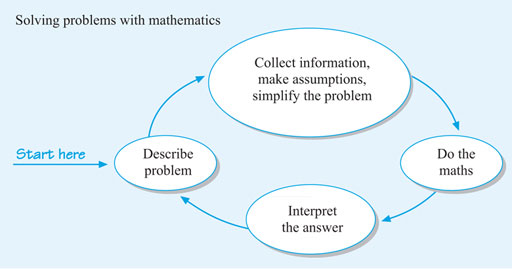Succeed with maths – Part 1

Start this free course now. Just create an account and sign in. Enrol and complete the course for a free statement of participation or digital badge if available.

Free course

# 7 Using maths in the real world

Quite often, you will need to decide how to tackle a problem, as well as considering what information you need. That can be a lot more challenging than working through some examples in a textbook.

However, it can be very useful to visualise problem solving as loop, in which you follow a series of steps to solve a problem. You can then repeat these if your answer needs further investigation or refining. We’re going to call this the maths cycle.

The steps in the cycle are summarised below.Figure _unit3.7.1 Figure 8 Mathematical modelling cycle

So you can see that the four main steps in this cycle:

• Describe the problem – do this as clearly as you can, remembering to discuss with others if you need to.
• Collect information and make any assumptions – making sure you have everything you need to solve the problem.
• Do the maths – deciding what mathematical techniques to use.
• Interpret the answer – so you know what this means practically and check that it is reasonable.

If the answer is not, you may need to refine the assumptions and go through the cycle again.

Now you’ll see how the maths cycle works in practice.